Subsections

# Interface Layer in Landau Theory

## Euler-Lagrange Equation

We need to minimize the functional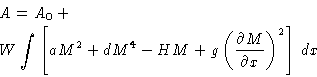with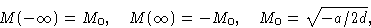This is a Lagrange problem with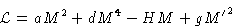On the coexistence line H=0 (Why?). We have: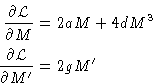and Euler-Lagrange equation becomes

 gM''=aM+2dM3 (6)

## Solution of Euler-Lagrange Equation

Multiply (6) by M'. Then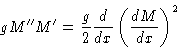and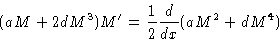Therefore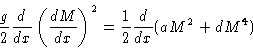and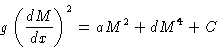To find C note that at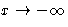we have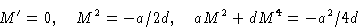We obtain: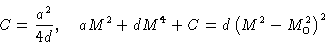This gives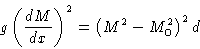or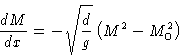(7)
and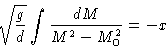Solution: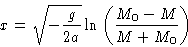(8)
This gives x(M). Inverting this function, we obtain density profile M(x)

Width of the interface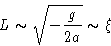(9)
The length of the interface is proportional to the correlation length!

## Surface Tension

In the bulk the energy per unit volume is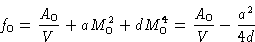In the interface layer we have free energy per unit volume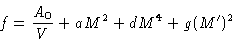Additional free energy per unit volume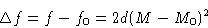Surface tension is surface energy per unit area, i.e.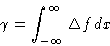Change of variables: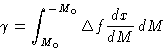or, substituting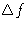and M'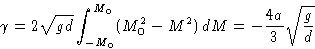(10)

## Critical Behavior

What happens at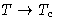? Coefficient a tends to zero as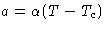.

From (9) and (10) we obtain: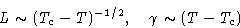As we are closer to the critical point, the interface layer becomes thicker, and the surface tension drops. Since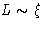, correlation length diverges, and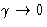means that the difference between the phases disappears. The penalty for forming interface becomes lower, and fluctuations grow.Next: Quiz Up: Spatial Inhomogeneity. Interfaces Previous: Mathematical Digression: Euler-Lagrange Equation

© 1997 Boris Veytsman and Michael Kotelyanskii
Thu Oct 16 20:58:44 EDT 1997